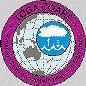Z-R Relations from Airborne Disdrometers

The least squares power law regression for the total sample of 14,254 6-s values gives an overall Z-R relation of Z=192R 01.42. This regression is dominated by the preponderance of stratiform precipitation. In a typical flight leg the plan view and vertical cross section of reflectivity shows the convective cells, the prominent bright band, and the large extent of stratiform precipitation. The same features are immediately evident in the in situ data profiles of <w> and R0.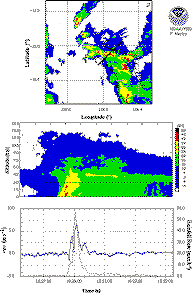In order to correct R0 for vertical air motions we use the relation

Rw/R0 = (<Vm> - <w>) / <Vm>

where <Vm> is the mass weighted mean fallspeed of the drop size distributions and <w> is the 6-s mean updraft, positive upwards. We have computed <Vm> from the relation R 0 = K <Vm> M, where M is the rain mass in the drop size distribution, and K is a constant that includes the sample volume and unit conversion (for a typical sample volume of 1,454 liters, K=2.5). Corrections have been made for the decreasing air density aloft. Clearly as <w> approaches <V m>, Rw approaches zero implying that the mass of rain is suspended. In fact, drops having fall speeds less than <w> rise, and conversely for the larger drops. This is analogous to the "balance level" for radar reflectivity where the mean Doppler velocity at vertical incidence is zero (Atlas, l966), except that <Vm> = <w>. This means that the balance level for Z usually occurs somewhat higher than that for M where the updrafts are stronger.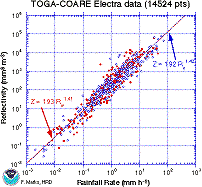The Z-R relations for these two distributions are nearly identical, due to the preponderance of points with <w> < ± 0.5 m/s (75%).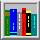References

Atlas, D., 1966: The balance level in convective storms. J. Atmos. Sci., 23, 635-651.

Atlas, D., P. Willis, and F. Marks, 1995: Draft effects upon reflectivity-rain rate relations. Proceedings of the 27th Conference on Radar Meteorology, Vail, CO, AMS.

Willis, P., R. Black, F. Marks, and D. Baumgardner, 1995: Airborne rain drop size distributions in TOGA COARE. Proceedings of the 21st Conference on Hurricanes and Tropical Meteorology, Miami, FL, AMS, 431-433.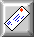marks@aoml.noaa.gov
Updated Thur, May 7, 1998